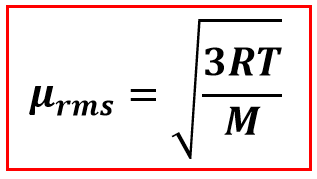# Problem: The rms (root-mean-square) speed of a diatomic hydrogen molecule at 50°C is 2000 m/s. Note that 1.0 mol of diatomic hydrogen at 50°C has a total translational kinetic energy of 4000 J.(A) Diatomic oxygen has a molar mass 16 times that of diatomic hydrogen. The root-mean-square speed vrms for diatomic oxygen at 50°C is:(B) The total translational kinetic energy of 1.0 mole of diatomic oxygen at 50°C is:(C) The temperature of the diatomic hydrogen gas sample is increased to 100°C. The root-mean-square speed vrms for diatomic hydrogen at 100°C is:   i. (16)(4000 J) = 64000 J ii. (4)(4000 J) = 16000 Jiii. 4000 Jiv. (1/4)(4000 J) = 1000 Jv. (1/16)(4000 J) = 150 Jvi. (√2)(2000 m/s) = 2800 m/s vii. (2)(2000 m/s) = 4000 m/s viii. (1/√2) (2000 m/s) = 1400 m/six. (1/2)(2000 m/s) = 1000 m/s

###### FREE Expert Solution

We are given for a diatomic Hydrogen, H2: its root-mean-square speed at 50°C is 2000 m/s, and the translational kinetic energy of 1 mole at 50°C is 4000 J.

Part A:

We are asked to calculate the root-mean-square speed (vrms) of diatomic oxygen which has a molar mass that is 16 times that of the diatomic hydrogen at 50°C.

Recall that the root-mean-square speed is the measure of the speed of particles in a gas, defined as the square root of the average velocity-squared of the molecules in gas

This is calculated as follows:Where:

μrms = rms speed

= 8.314 J/mol∙K

= Temperature, Kelvin

M = molar mass, kg/mol

Given:

T = 50°C

(Convert to Kelvin)

T = 50 + 273.15

T = 323.15 K

92% (142 ratings)###### Problem Details

The rms (root-mean-square) speed of a diatomic hydrogen molecule at 50°C is 2000 m/s. Note that 1.0 mol of diatomic hydrogen at 50°C has a total translational kinetic energy of 4000 J.

(A) Diatomic oxygen has a molar mass 16 times that of diatomic hydrogen. The root-mean-square speed vrms for diatomic oxygen at 50°C is:

(B) The total translational kinetic energy of 1.0 mole of diatomic oxygen at 50°C is:

(C) The temperature of the diatomic hydrogen gas sample is increased to 100°C. The root-mean-square speed vrms for diatomic hydrogen at 100°C is:

i. (16)(4000 J) = 64000 J

ii. (4)(4000 J) = 16000 J

iii. 4000 J

iv. (1/4)(4000 J) = 1000 J

v. (1/16)(4000 J) = 150 J

vi. (√2)(2000 m/s) = 2800 m/s

vii. (2)(2000 m/s) = 4000 m/s

viii. (1/√2) (2000 m/s) = 1400 m/s

ix. (1/2)(2000 m/s) = 1000 m/s